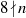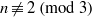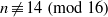Hostname: page-component-758b78586c-qvhcc Total loading time: 0 Render date: 2023-11-28T16:01:29.694Z Has data issue: false Feature Flags: { "corePageComponentGetUserInfoFromSharedSession": true, "coreDisableEcommerce": false, "useRatesEcommerce": true } hasContentIssue false

# ON WARING’S PROBLEM: TWO SQUARES AND THREE BIQUADRATES

Published online by Cambridge University Press:  28 June 2013

## Abstract

We investigate sums of mixed powers involving two squares and three biquadrates. In particular, subject to the truth of the Generalised Riemann Hypothesis and the Elliott–Halberstam conjecture, we show that all large natural numbers$n$ with$8\nmid n$,$n\not\equiv 2~(\text{mod} ~3)$ and$n\not\equiv 14~(\text{mod} ~16)$ are the sum of two squares and three biquadrates.

## MSC classification

Type
Research Article
Information
Mathematika , January 2014 , pp. 153 - 165

## Access options

Get access to the full version of this content by using one of the access options below. (Log in options will check for institutional or personal access. Content may require purchase if you do not have access.)

## References

Brüdern, J., Sums of squares and higher powers. J. Lond. Math. Soc. (2) 35 (1987), 233243.Google Scholar
Brüdern, J., A problem in additive number theory. Math. Proc. Cambridge Philos. Soc. 103 (1988), 2733.Google Scholar
Davenport, H., Analytic Methods for Diophantine Equations and Diophantine Inequalities, 2nd edn. Cambridge University Press (Cambridge, 2005).Google Scholar
Dietmann, R. and Elsholtz, C., Sums of two squares and one biquadrate. Funct. Approx. Comment. Math. 38 (2008), 233234.Google Scholar
Duke, W., Friedlander, J. B. and Iwaniec, H., Weyl sums for quadratic roots. Int. Math. Res. Not. 2012 (11) (2012), 24932549; Corrigendum, Int. Math. Res. Not. 2012(11) (2012), 2646–2648.Google Scholar
Friedlander, J. B. and Iwaniec, H., Opera de Cribro, American Mathematical Society (Providence, RI, 2010).Google Scholar
Gauss, C. F., Disquisitiones Arithmeticae (Leipzig, 1801).Google Scholar
Golubeva, E. P., On nonhomogeneous Waring equations, Zap. Nauchn. Sem. S.-Peterburg. Otdel. Mat. Inst. Steklov. (POMI) 226 (1996), Anal. Teor. Chisel i Teor. Funktsii. 13, 65–68, 236; translation in J. Math. Sci. (New York) 89 (1998), 955–957.Google Scholar
Golubeva, E. P., A bound for the representability of large numbers by ternary forms, and nonhomogeneous Waring equations, Zap. Nauchn. Sem. S.-Peterburg. Otdel. Mat. Inst. Steklov. (POMI) 357 (2008), Anal. Teor. Chisel i Teor. Funktsii. 23, 5–21, 224; translation in J. Math. Sci. (New York) 157 (2009), 543–552.Google Scholar
Hooley, C., On a new approach to various problems of Waring’s type. In Recent Progress in Analytic Number Theory, Vol. 1 (Durham, 1979), Academic Press (London–New York, 1981), 127191.Google Scholar
Hooley, C., On Waring’s problem for two squares and three cubes. J. Reine Angew. Math. 328 (1981), 161207.Google Scholar
Hooley, C., On Waring’s problem for three squares and an lth power. Asian J. Math. 4 (2000), 885903.Google Scholar
Kawada, K. and Wooley, T. D., Sums of fourth powers and related topics. J. Reine Angew. Math. 512 (1999), 173223.Google Scholar
Kawada, K. and Wooley, T. D., Davenport’s method and slim exceptional sets: the asymptotic formulae in Waring’s problem. Mathematika 56 (2010), 305321.Google Scholar
Linnik, Yu. V., Additive problems involving squares, cubes and almost primes. Acta Arith. 21 (1972), 413422.Google Scholar
Vaughan, R. C., On sums of mixed powers. J. Lond. Math. Soc. (2) 3 (1971), 677688.Google Scholar
Vaughan, R. C., On Waring’s problem for smaller exponents. Proc. Lond. Math. Soc. (3) 52 (1986), 445463.Google Scholar
Vaughan, R. C., The Hardy–Littlewood Method, 2nd edn. Cambridge University Press (Cambridge, 1997).Google Scholar